Mobile QR Code1. (Department of System Semiconductor Engineering, Sangmyung University, Korea)
2. (Department of Electronic Engineering, Inha University, Incheon 22212, Korea)

Dual-mode, PWM, PFM, digital dual-mode controller, DC-DC converter

## I. INTRODUCTION

Portable devices such as smartphones and wearable systems have widely incorporated artificial intelligence techniques, which consume a large amount of computation power over application processors (APs) and microprocessors (MPUs). To decrease the power consumption, portable devices have been equipped with a power management integrated circuit (PMIC) based on a switching mode power supply (SPMS) with a high power efficiency . A DC-DC converter for PMICs on AP and MPU within portable devices should be capable of managing a broad current load range between a few $\mu$A to a few hundred mA.

To this end, pulse frequency modulation (PFM) for a light current load [2,3] and pulse width modulation (PWM) for a heavy current load [4,5] have been widely employed to avoid a poor conduction loss and switching loss, hereby achieving a high power efficiency. However, these DC-DC converters have still suffered from a poor power efficiency in the transit time between PFM and PWM over the system due to inaccurate control methodologies. Manual control techniques with independent PWM and PFM circuits, which have been used to facilitate the transition between the PWM and PFM modes correspond to slow transit time and poor power efficiency . Moreover, the current sensing methods to monitor the voltage drop across series resistors [7,8] or power transistors  are sensitive to noise and matching problems in the layout. The employment of an error amplifier as a PFM signal detection circuit enabled the DC-DC converter to change the PFM mode to the PWM mode [10,11]. However, this technique cannot accurately detect a heavy load current signal, resulting in a higher switching frequency of the PFM DC-DC converter and a low power efficiency. Another weakness of this method was incapable of handling a fast transient current load. Recently, dual-model DC-DC buck converters were implemented to cover both light current load and heavy current load for mobile applications [12-16]. Multiple-sawtooth waveform technique  was employed to include light and heavy current load. This technique demonstrated excellent performance on PWM mode, but poor performance on PFM mode. Several seemless switching techniques [13-16] based on the pre-determined threshold current set were implemented to make transition between PFM mode and PWM mode. These techniques enforced PMIC users manually to set pre-determined boundary current between two modes. Considering these aspects, this paper proposes a dual-mode DC-DC converter that can ensure a smooth transition between the PFM and PWM modes with multi-bit control technique, and maintain a competitive power efficiency for the dual-mode.

The remaining paper is organized as follows. Section II describes the design technique of the mode controller and DC-DC control circuit based on 3-bit multiplexer. The measurement results are discussed in Section III. Section IV presents the concluding remarks.

## II. PROPOSED ARCHITECTURE

The proposed architecture includes a PFM controller, a PWM controller, a PFM/PWM mode controller, a dead time controller, gate drivers, a soft start circuit, and a bandgap reference within the on-chip, as shown in Fig. 1.

The off-chip circuit consists of an inductor (L), an output capacitor ($C_{out}$), a resistive feedback circuit ($R_{u}$ and $R_{d}$), and a current load (load). The off-chip resistive feedback resistors are solely utilized for the measurement of power efficiency with the external load current. The PFM/PWM controller receives two signals: a feedback signal $V_{fb}$ from the resistive feedback circuit, and a voltage reference signal $V_{ref}$ from the bandgap reference circuit and soft start circuit. The PFM/PWM DC-DC controller produces duty signals such as PFM_D and PWM_D if the enable signal (PFM_EN, PWM_EN) from the PFM/PWM mode controller becomes high. The PFM/PWM mode controller is driven by three bits $S_{i}$, where i can be 1, 2, or 3, and this controller generates the duty signal, Duty. The duty signal drives both the gate drivers and dead time control circuit. The zero current detector sensing $V_{x}$ from the inductor (L) produces a signal pertaining to the dead time detection, which is fed to the dead time control circuit to control two signals, NSIG and PSIG, and prevent two power switches ($M_{P}$,$~ M_{N}$) from simultaneously switching on. The feedback signals from the resistive feedback circuit inductor, output capacitor, resistor feedback circuit, and load are enclosed in the off-chip circuit.

The PFM DC-DC controller in the proposed circuit is presented in Fig. 2. The output signal $V_{comp}$ from a hysteresis comparator with a trip voltage greater than 100~mV drives four transistors (two MNPDs and two MPPDs). The reference current $I_{ref}$ is provided by the bandgap reference circuit. Employment of the bandgap reference circuit allows the proposed circuit to become robust against PVT variation. In addition, the 3-bit digital control circuit offers the degree of freedom to change the boundary current between PFM mode and PWM mode with 3-bit resolution, so that the boundary current change due to PVT variation can be minimized by varying the digital status of the 3-bit digital control circuit.

The capacitor, $C_{os}$ and MN2 generates a sawtooth waveform to drive the combinational logic circuits, namely, three inverters, a NOR gate, an AND gate, and a SR flip-flop. The simulated waveforms, including Vos, D, Set, and Reset, are illustrated in Fig. 3.

As D remains high to turn on the power switch, MN2 turns off and $C_{os}$ is gradually charged to generate the sawtooth waveform. The turn-on time ($T_{on}$) of the power switch in the PFM controller is defined as

##### (1)
$T_{on}=~ \frac{C_{os}V_{thn}}{I_{ref}}$

where $V_{thn}$is the threshold voltage of MN2. $T_{on}$ remains constant because $C_{os}$, $V_{thn}$, and $I_{ref}$are invariant parameters. However, the switching frequency of the PWM controller varies with the load current.

The circuit schematic of the PWM DC-DC controller is shown in Fig. 4. Depending on clk and $\overline{clk}$, the switches (M2 and M8) may turn on or off. For example, if clk and $\overline{clk}$ are high and low, respectively, M2 and M8 turn on and off, and thus, $V_{c1}$ and $V_{c2}$ become high and low, respectively. Hence capacitors, $C_{1}$ and $C_{2}$ are charged and discharged, such that S and R of the SR flip-flop are low and high, respectively. This makes clk and $\overline{clk}$ become low and high. This cycle is repeated, as shown in Fig. 5, to generate the PWM control signals clk and $\overline{clk}$, which drive the power switches. The ratio of the slew rate of the sawtooth waveforms $V_{c1}$and $V_{c2}$ is simulated to be 1:9 because the ratio of $C_{1}$to $C_{2}~$is set as 1:9.

The turn-on time ($T_{on}$) of the power switch in the PWM controller can be specified as

##### (2)
$T_{on}=~ \frac{C_{1}}{I_{ref}}~ \frac{V_{IN}}{2}$

where $C_{1}$ and $V_{IN}$ denote the PWM capacitor for $V_{c1}$ and the input voltage, respectively.

The turn-off time ($T_{off}$) of the power switch in the PWM controller can be defined as

##### (3)
$T_{off}=~ \frac{C_{2}}{I_{ref}}~ \frac{V_{IN}}{2}$

where $C_{2}$ is the PWM capacitor for $V_{c2}$.

The total period time ($T_{total}$) of the PWM switching frequency ($f_{sw}$) becomes

##### (4)
$f_{sw}=\frac{1}{T_{total}}=~ \frac{2I_{ref}}{V_{IN}\left(C_{1}+C_{2}\right)}~$

The ratio of $C_{1}$ to $C_{2}$ is 1:9 in this design to generate the PWM signal of 2 MHz. Since PWM controller does not employ the analog compensation scheme, it may suffer from output ripple voltage in case of heavy load condition.

The power efficiency of the PFM and PWM DC-DC converters is usually a function of the load current, as shown in Fig. 6. As the load current varies, the power efficiency of the DC-DC converter is influenced by the conduction loss and switching loss. The power efficiency of the PFM DC-DC converter is higher than that of the PWM DC-DC converter under a low light load current (0 - $I_{min})$ and deteriorates as the load current increases because the switching loss becomes predominant. In contrast, the power efficiency of the PWM DC-DC converter is higher than that of PFM DC-DC converter under a heavy load current ($>I_{max})$ and deteriorates as the load current decreases because the conduction loss becomes predominant. The boundary load current ($I_{b}$) at which the power efficiency of the PFM and PWM modes is identical, varies, depending on the process and load conditions.

To ensure a smooth transition from one mode to the other mode, the minimum ($I_{min}$) and maximum loads ($I_{max}$) around the boundary load ($I_{b}$) should be defined by the feedback network which includes $R_{u}~$and $R_{d}$. The difference between $I_{min}$ and $I_{max}$ is divided by M (=$2^{N}$) to ensure that each load current, $I_{x}$ can be mapped to each time $t_{x}$, as presented in Fig. 7, where N is the number of bits of the counter.

This timing diagram can be implemented by a N-bit counter. The 5-bit counter was employed in this design with 3-bit ($S_{1},S_{2},S_{3}$) multiplexer circuit and a slow clock signal (SCLK) to determine $t_{x}$ (time of changing one mode to another), as shown in Fig. 8.

The PFM/PWM dual-mode controller shown in Fig. 8 consists of a 5-bit counter (C0, C1, {\ldots}, C31), a D-flip/flop, an OR gate, and three multiplexers. As the load current, $I_{1}$ in the PFM mode becomes $2I_{1}$, sensed by the feedback circuit shown in Fig. 9, the DC-DC converter remains in the PFM mode with the switching frequency $f_{sw}$, which increases as indicated in (5)

##### (5)
$f_{sw}=\frac{I_{load}}{2\pi C_{out}V_{out}}\left(1-\frac{V_{out}}{V_{in}}\right)$

As the PFM_EN signal is implemented, owing to the zero current detecting signal (ZC), the 5-bit counter begins to count until the counter number ($N_{x}$) reaches the number ($C_{i}$) set by the 3-bit multiplexer circuit ($S_{1},S_{2},S_{3}$), where $C_{i}$ is specified as

##### (6)
$C_{i}=2^{2}S_{1}+2^{1}S_{2}+2^{0}S_{3}$

As soon as $N_{x}$ reaches $C_{i}$, the dual-mode controller allows the DC-DC converter to change from one mode to the other mode. As the load current decreases from 250~mA (PWM mode) to 10 mA (PFM mode) with $C_{i}$ set as 25, the counter number ($N_{x}$) from the zero current detection signal reaches $C_{i}$ of 25. Moreover, the DC-DC converter remains in the PFM mode as the output voltage ($V_{out}$) remains constant, as indicated by the simulation result shown in Fig. 10. In this manner, the PFM/PWM dual-model controller is capable of setting $t_{x}$ through $C_{i}$ associated with the 5-bit counter and 3-bit multiplexer circuit.

##### Fig. 1. Block diagram of the proposed dual mode DC-DC converter with a digital mode controller.}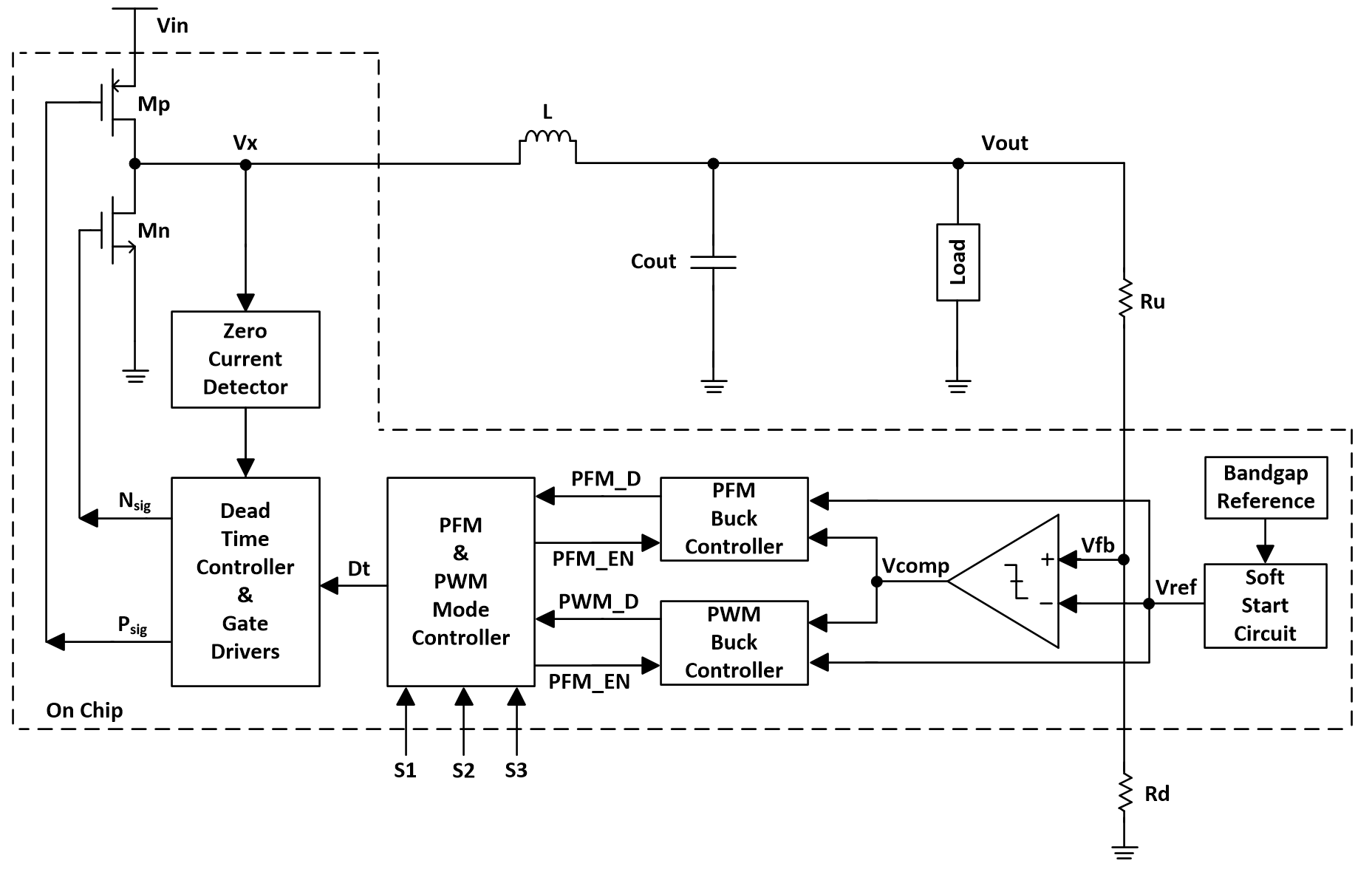##### Fig. 2. Circuit schematic of the PFM controller.}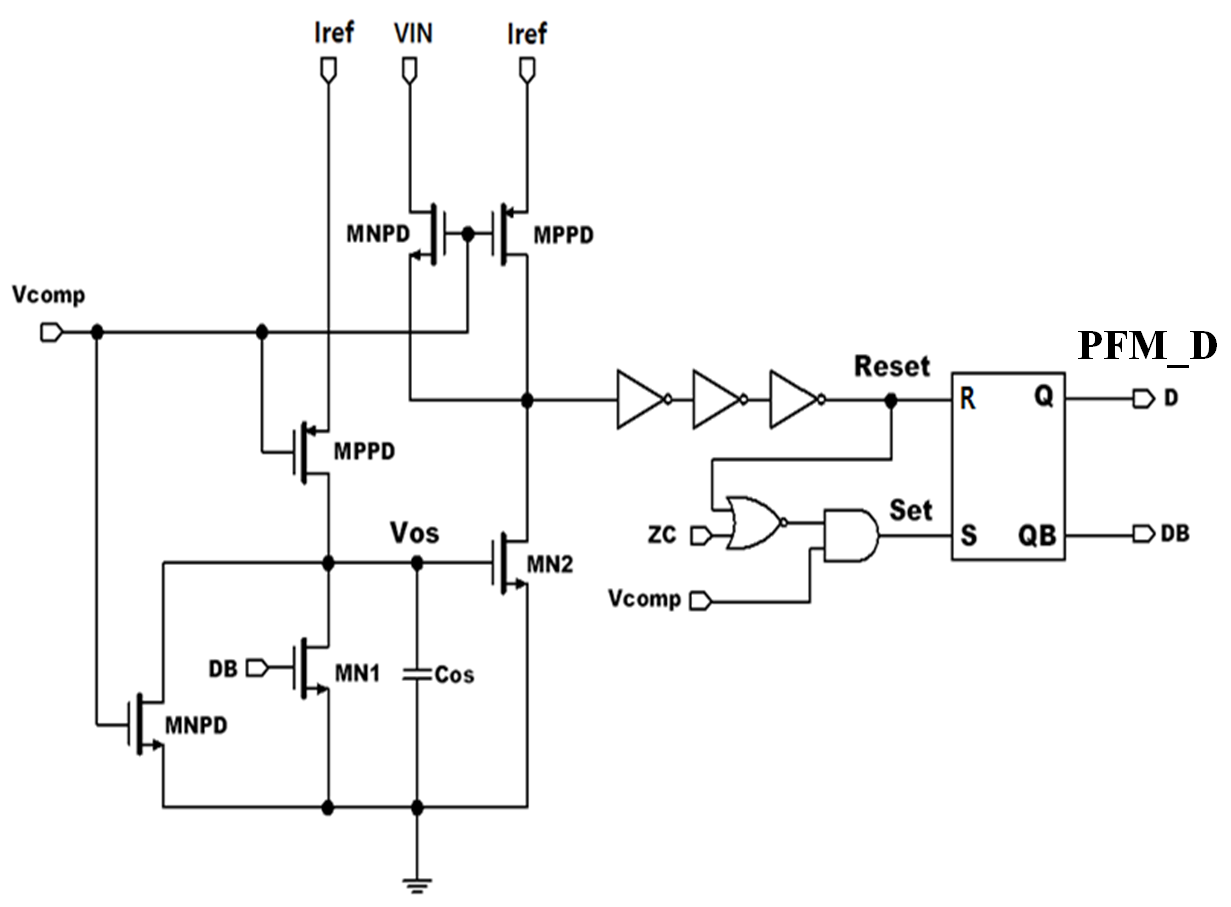##### Fig. 3. Simulated waveforms of the PFM controller.}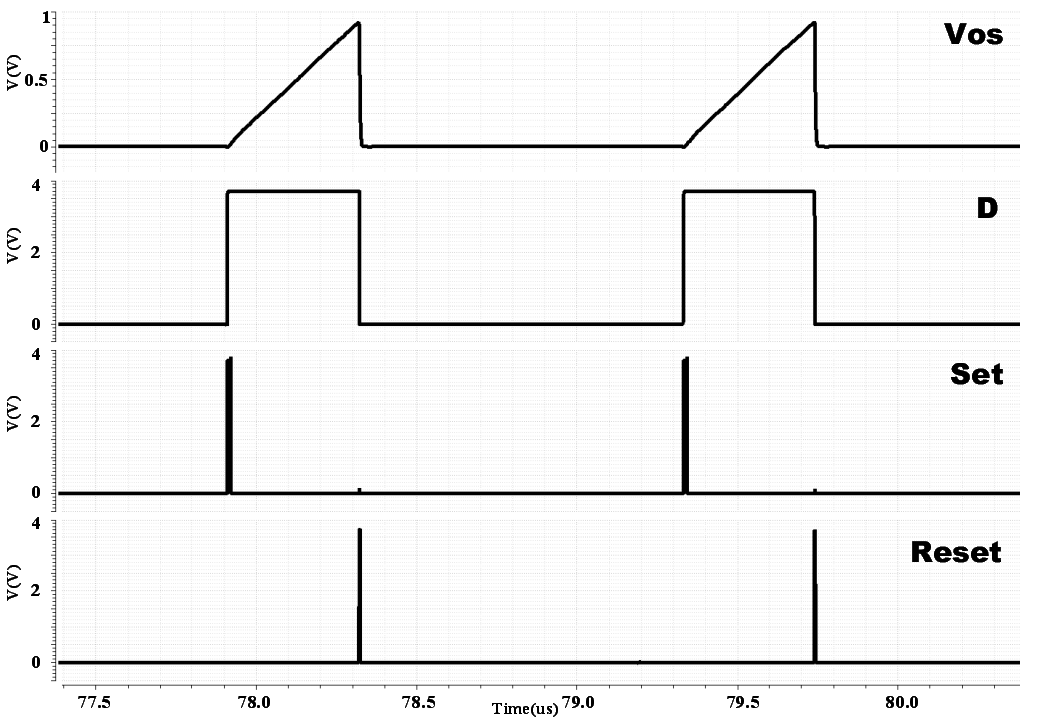##### Fig. 4. Circuit diagram of the PWM controller.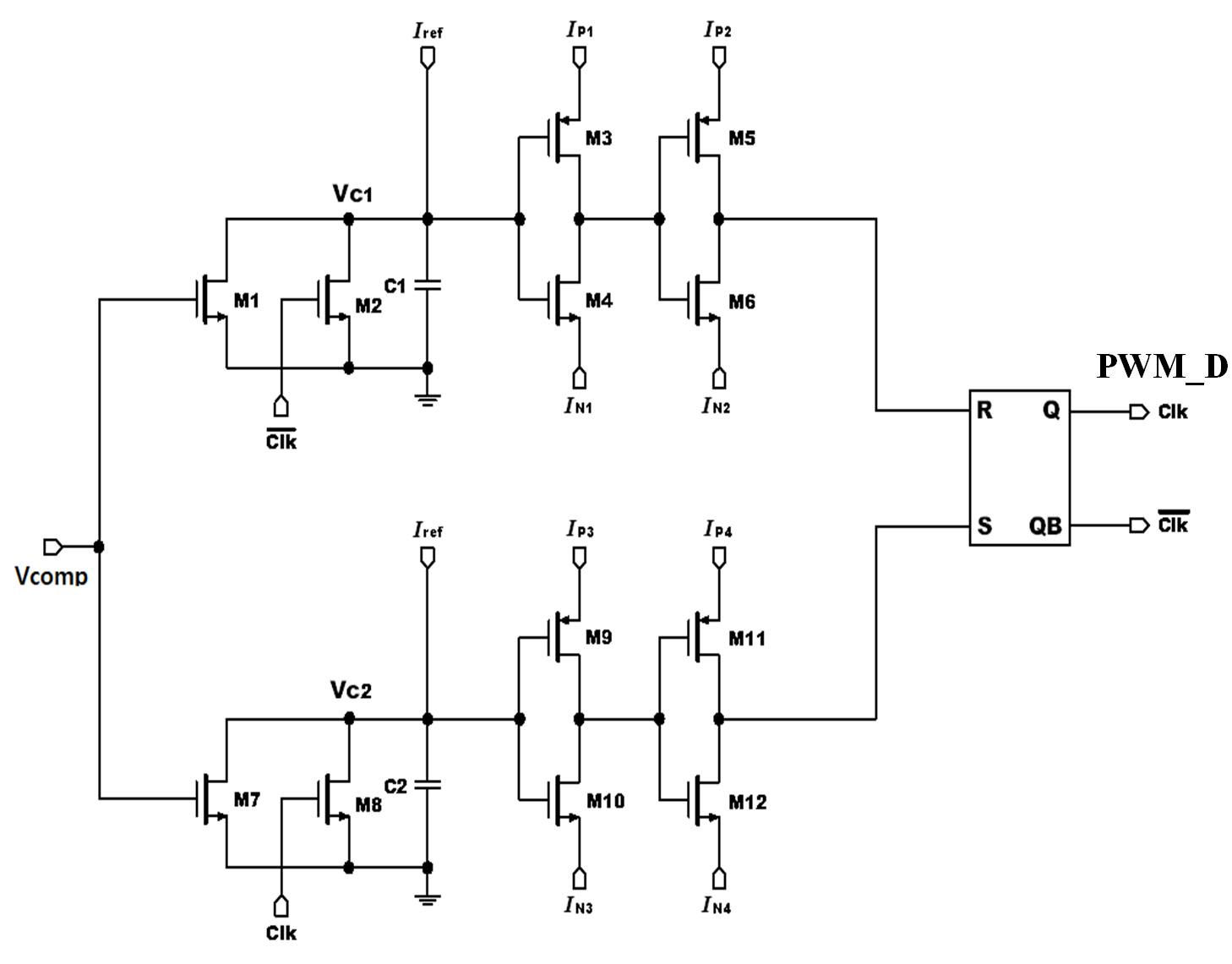##### Fig. 5. Simulated waveforms of the PWM controller.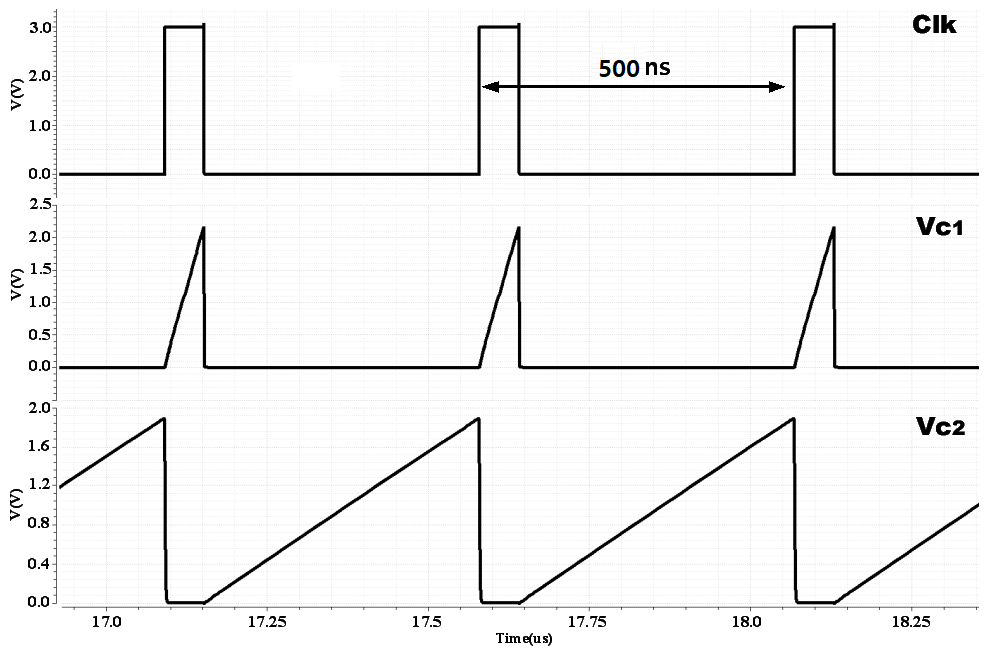##### Fig. 6. Power efficiency of the PFM and PWM modes as a function of the load current.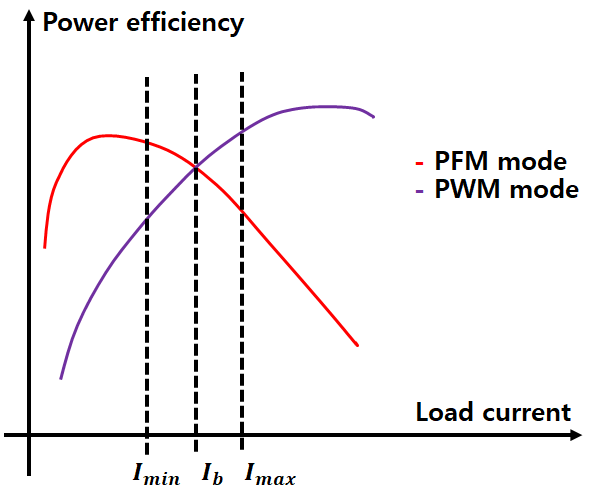##### Fig. 7. Timing diagram associated with the load current.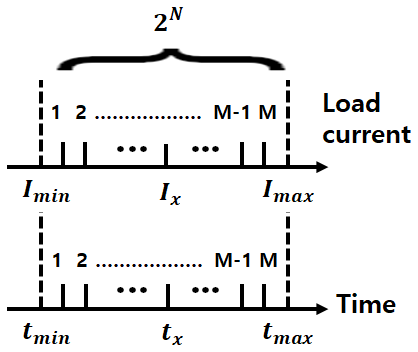##### Fig. 8. Block diagram of the PFM/PWM dual-mode controller.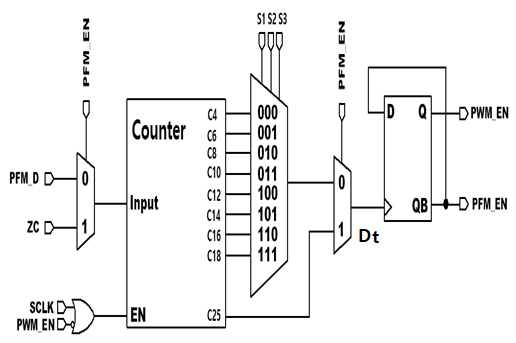##### Fig. 9. Timing diagram of the dual-mode controller.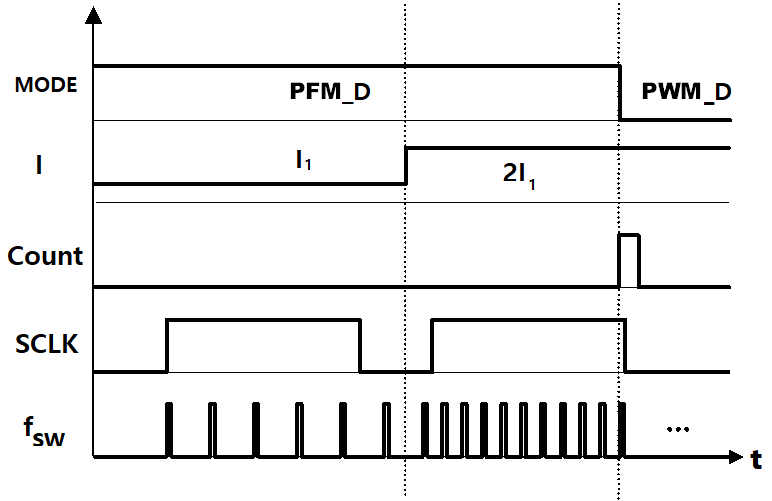##### Fig. 10. Simulated waveforms of the output voltage ($V_{out}$), inductor current (I), counter $(N_{x}$), and load current ($I_{load}$).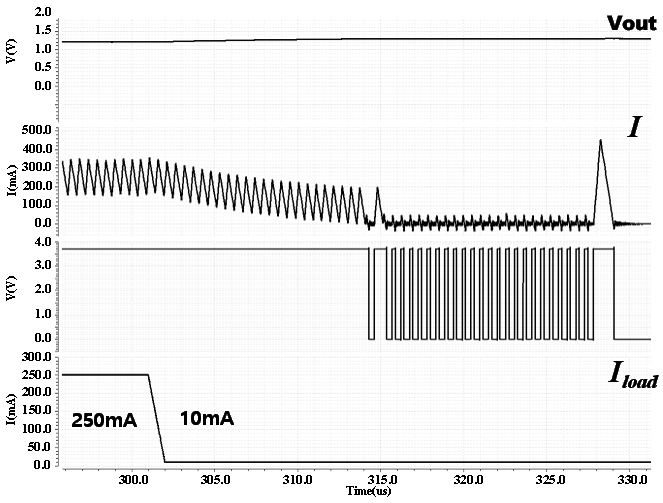## III. MEASUREMENT RESULTS

The proposed dual-mode DC-DC converter with a digital dual mode controller is implemented through a 180 nm BCDMOS process. The threshold voltage and on-resistance of the power switches are 0.6 V and 70 m${\Omega}$, respectively. The micro-photograph of the proposed circuit is shown in Fig. 11. The active chip area occupies 1760 ${\mu}$m ${\times}$ 1530 ${\mu}$m, excluding the bonding pads.

Analog blocks, such as the band gap reference (BGR) and inductor current sensor circuit, are placed on the bottom of the chip layout, such that they are isolated from the digital blocks. In addition, to prevent digital noise from the digital blocks, the digital blocks such as the PFM controller, PWM controller, and dual-mode controller are placed on top of the chip. Placement of two power switches and gate drivers is made on the right side of the chip layout.

The photograph of the print circuit board shown in Fig. 12 illustrates the proposed chip on board, inductor, bypass capacitors, digital buffers, 3-bit control switch, circled wire to measure the inductor current, and power connectors.

The photograph of the proposed circuit within the chip test jig in the testing environment, including the current prober(Agilent DC electronic load, 6060B), digital oscilloscope(Agilent DSO7174B), function generator (Agilent 33210A) and power supply (Agilent E 3630) is shown in Fig. 13.

To examine the dual-mode functionality of the proposed circuit with a digital controllability, the boundary current ($I_{b}$) between the PFM and PWM modes is set as 100 mA with the 3-bit control signal of $C_{i~ }$set as 4, 10, and 18. As the load current increases from 0 A to 200 mA with $C_{i~ }$set as 4, the measured waveforms of the output voltage ($V_{out}$), duty clock, inductor current ($I_{L}$), and load current ($I_{load}$) are as illustrated in Fig. 14. The duty clock signal and inductor current signal with a control signal of four pulses are measured, so that the dual mode controller is under the control ($C_{i~ }$) of 3-bit multiplexer circuit. The switching frequency of the output voltage, duty clock, and inductor current waveforms is shown to be increasing to 2 MHz as the load current changes.

Similar measurements are performed with $C_{i~ }$set as 10 and 18. The duty clock signals and inductor current signals with control signal of 10 pulses are measured, as shown in Fig. 15. As the control signal of 10 pulses is applied to the proposed circuit with the load current increasing, the frequency of duty clock signal and output voltage is increased to 2.1 MHz. The output ripple voltage is measured to be approximately 30 mV.

As the control signal of 18 pulses is applied to the proposed circuit with the load current increasing, the frequency of duty clock signal and output voltage is increased to 2.0 MHz. The output ripple voltage is measured to be approximately 25 mV

As the load current decreases from 415 mA to 0 mA with 3-bit control signal of $C_{i~ }$set as 16, the measured waveforms of the output voltage ($V_{out}$), duty clock, inductor current ($I_{L}$), and load current ($I_{load}$) are as illustrated in Fig. 17. The 16 control pulses can be clearly seen from the inductor current. The switching frequency of PWM with the load current is measured to be 2.2 MHz and output ripple voltage is shown to be 25 mV.

These four measurement results with 3-bit control signal ($C_{i~ }$) illustrate the functionality of 3-bit digital mode controller capable of controlling the number of control pulse and changing the position of the boundary current between PFM mode and PWM mode.

The power efficiencies of the proposed dual-mode DC-DC converter are measured under various load currents ranging from 10 mA to 500 mA, as presented in Fig. 18. The measured power efficiency graph include three different measurement data such as power efficiency of PFM mode, PWM mode, and dual mode operation. The power efficiency of the dual mode operation with the boundary current (90 mA) covers PFM mode in the light current load (10-90 mA) and PWM mode in the heavy current load (90-500 mA).

The PWM and PFM measurement results of the proposed dual mode DC-DC converter show that the maximum power efficiencies reach 91 % and 85% at 180 mA and 80mA, respectively. The measured power efficiency of the PWM mode is the same as that of the PFM mode at a load current of 90 mA. Transition between the PFM and PWM modes is demonstrated by setting the boundary load current ($I_{b}$) of 90 mA.

Table 1 presents a performance comparison of the proposed DC-DC converter and conventional converters. The input/output voltage range, switching frequency range, and output ripple voltage of the proposed dual-mode DC-DC converter are comparable with those of the conventional ones. The proposed converter offers the competitive power efficiency with wider load current range, less switching frequency range, and less output ripple voltage than the conventional ones. While the conventional ones incorporate the predetermined threshold current manually to drive dual-mode operation, the proposed converter employs the 3-bit PFM/PWM dual-mode digital controller to make a smooth transit between two modes. Measurement results demonstrate that the proposed converter is superior to the conventional ones with respect to PFM/PWM power efficiency, output ripple voltage, and 3-bit digital mode control technique.

##### Table 1. The performance comparison of the proposed dual-mode DC-DC converter and conventional converters
 Parameter    This work Technology 180 nm 65 nm 130 nm 180 nm Input voltage (V) 2.0-3.3 1.8 2.2-3.3 2.2-5.0 Output voltage (V) 1.2 0.5-1.5 1.7 1.2 Inductor (µH) 1 0.22 3.0 2.2 Capacitor (µF) 2.2 4.7 3 22 Switching frequency (MHz) 0.13-7.4 10 0.15-2.5 0.06-2.3 Ripple voltage (mV) 40-153 40-150 180 12-32 Load range (mA) 3-200 2-800 0.01-20 10-500 PFM/PWM efficiency (%) 82(91) 80(90) 81(92) 85(91) Mode control circuit Predetermine current manually Predetermine current manually Predetermine current manually 3-bit digital dual-mode control
##### Fig. 11. Chip micro-photograph of the proposed circuit.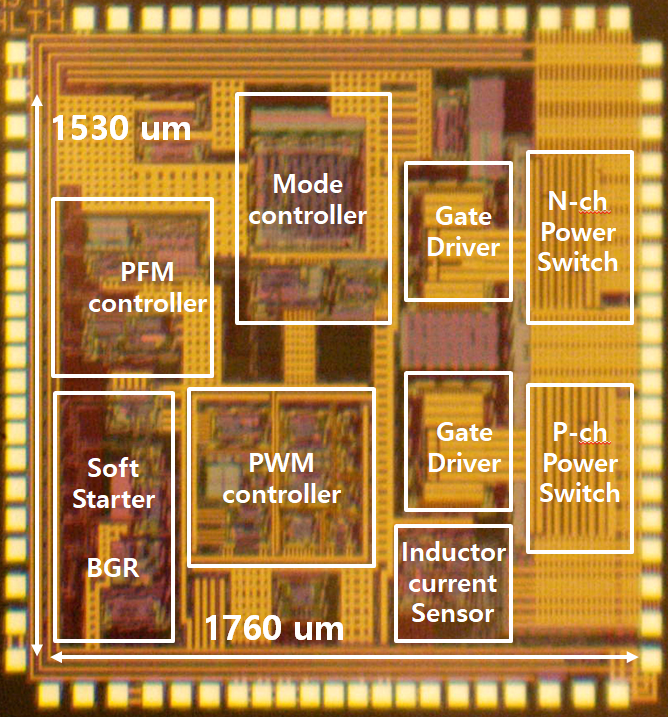##### Fig. 12. Photograph of the print circuit board.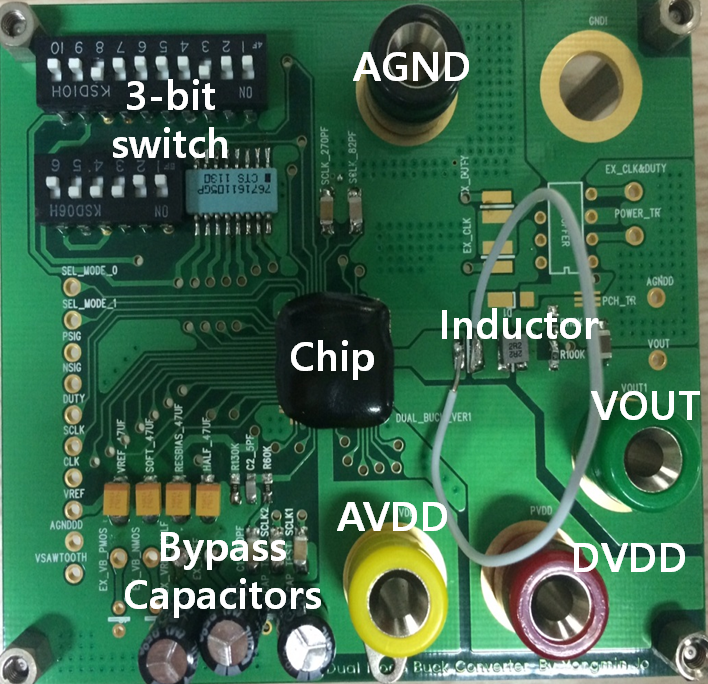##### Fig. 13. The photograph of the proposed circuit with the testing environment.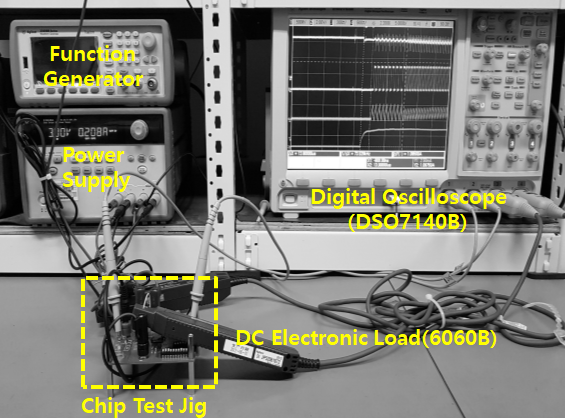##### Fig. 14. Measured waveforms of the output voltage ($V_{out}$), duty clock, inductor current ($I_{L}$), and load current ($I_{L}$) with 3-bit control signal of 000($C_{i}$ set as 4).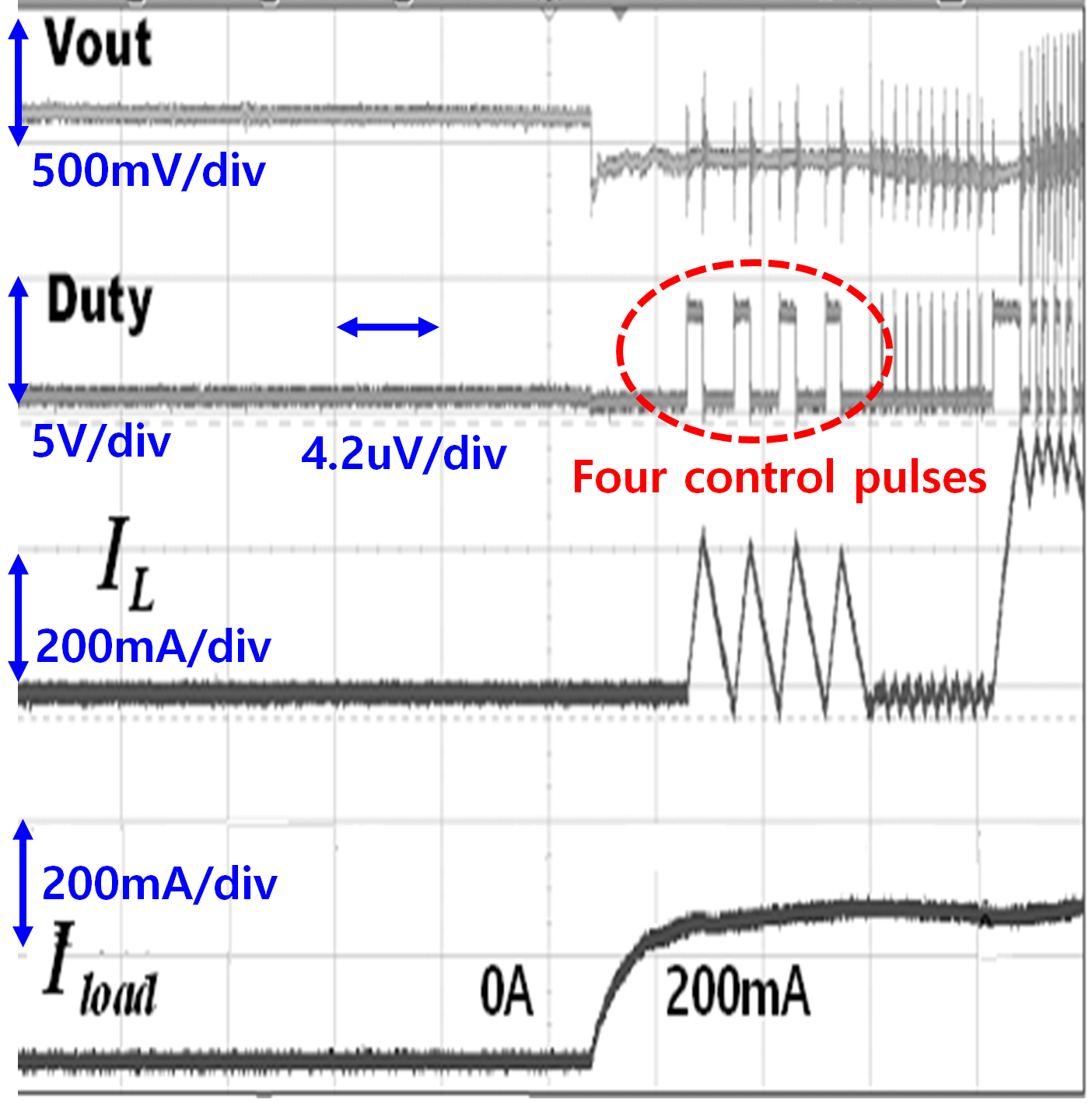##### Fig. 15. Measured waveforms of the output voltage ($V_{out}$), duty clock, inductor current ($I_{L}$), and load current ($I_{load}$) with 3-bit control signal of 011($C_{i}$ set as 10).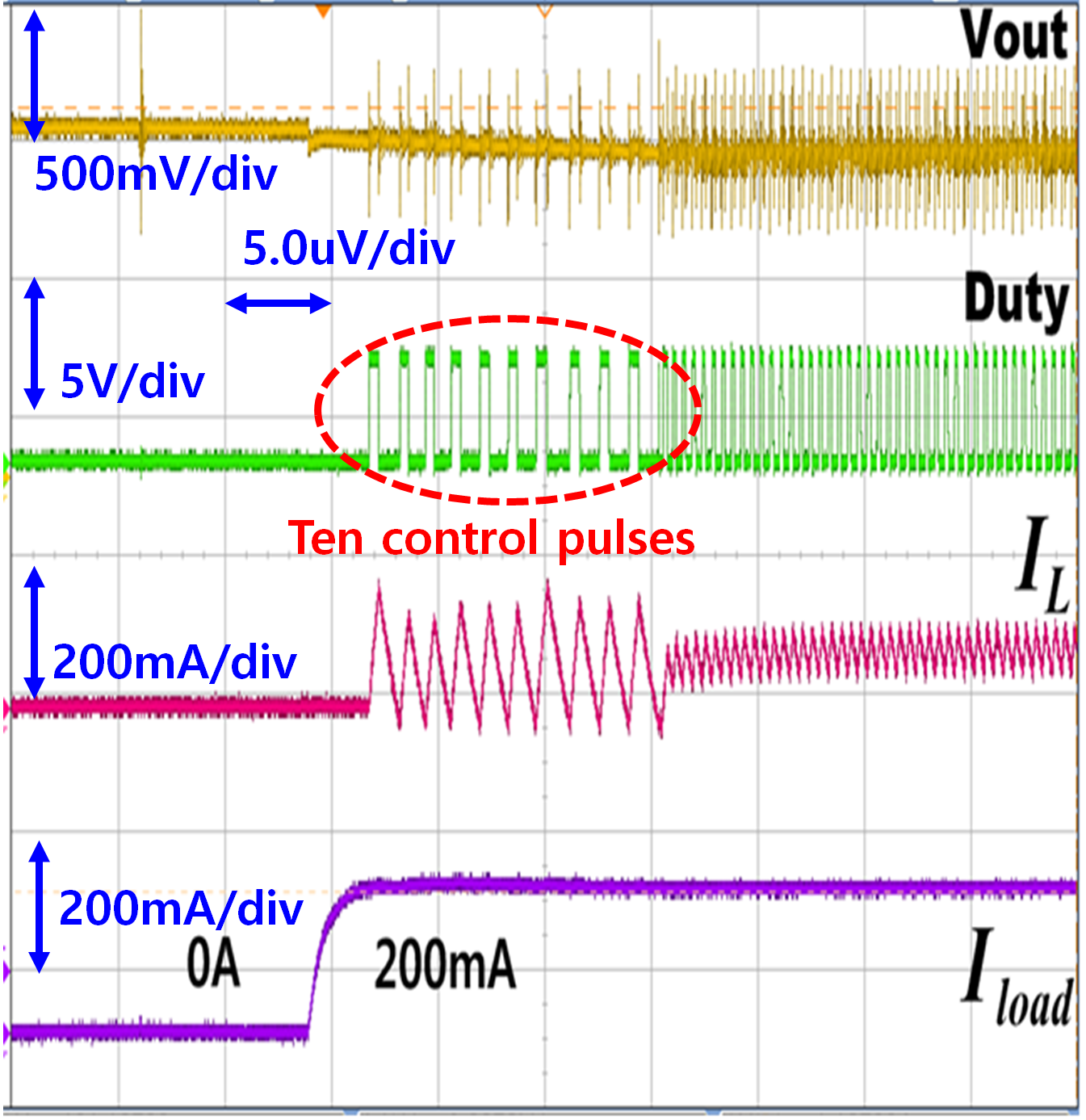##### Fig. 16. Measured waveforms of the output voltage ($V_{out}$), duty clock, inductor current ($I_{L}$), and load current ($I_{load}$) with 3-bit control signal of 111($C_{i}$ set as 18).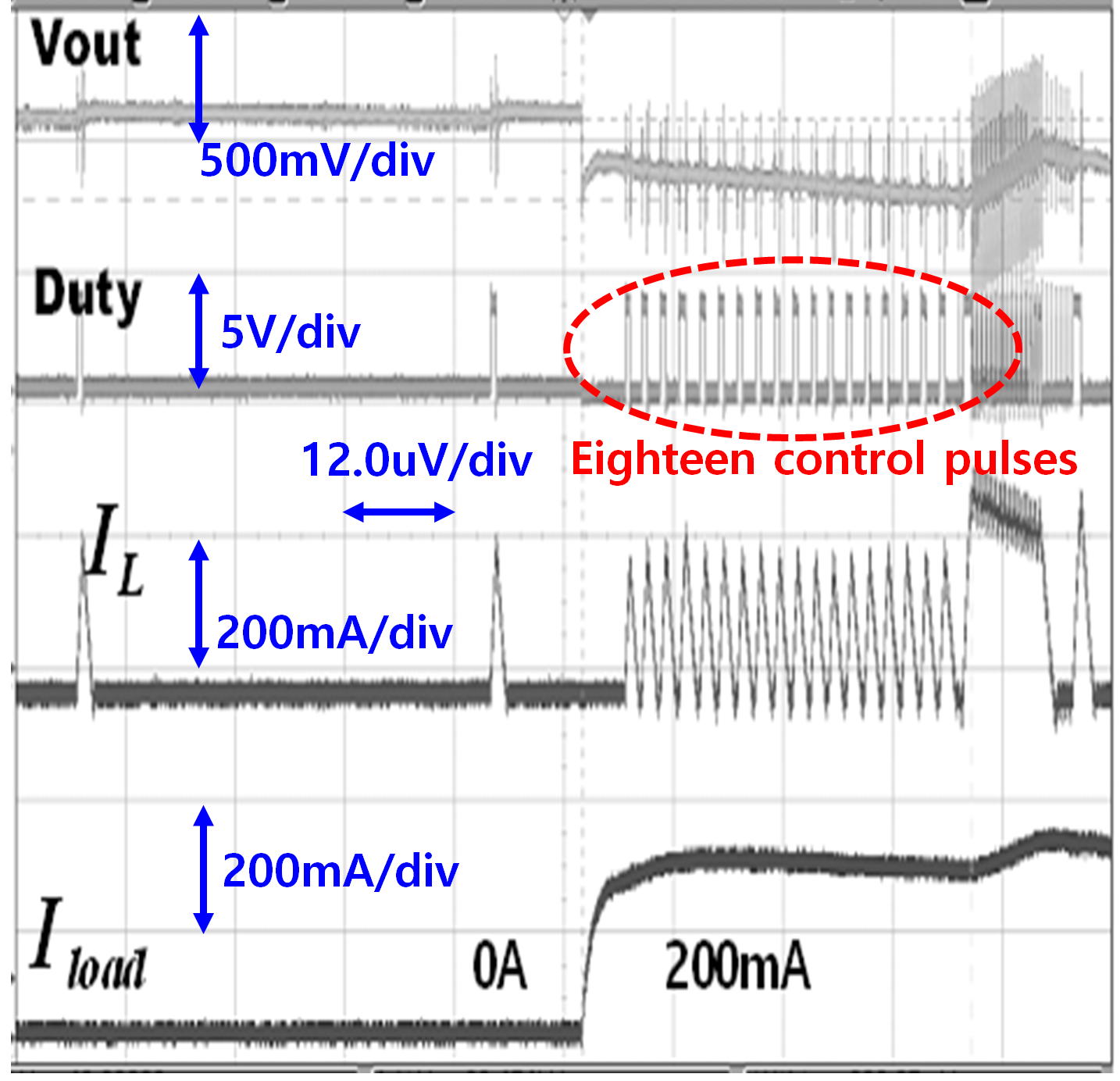##### Fig. 17. Transient measurement result from the PWM mode to PFM mode with 3-bit signal of 110 ($C_{i}$ set as 16).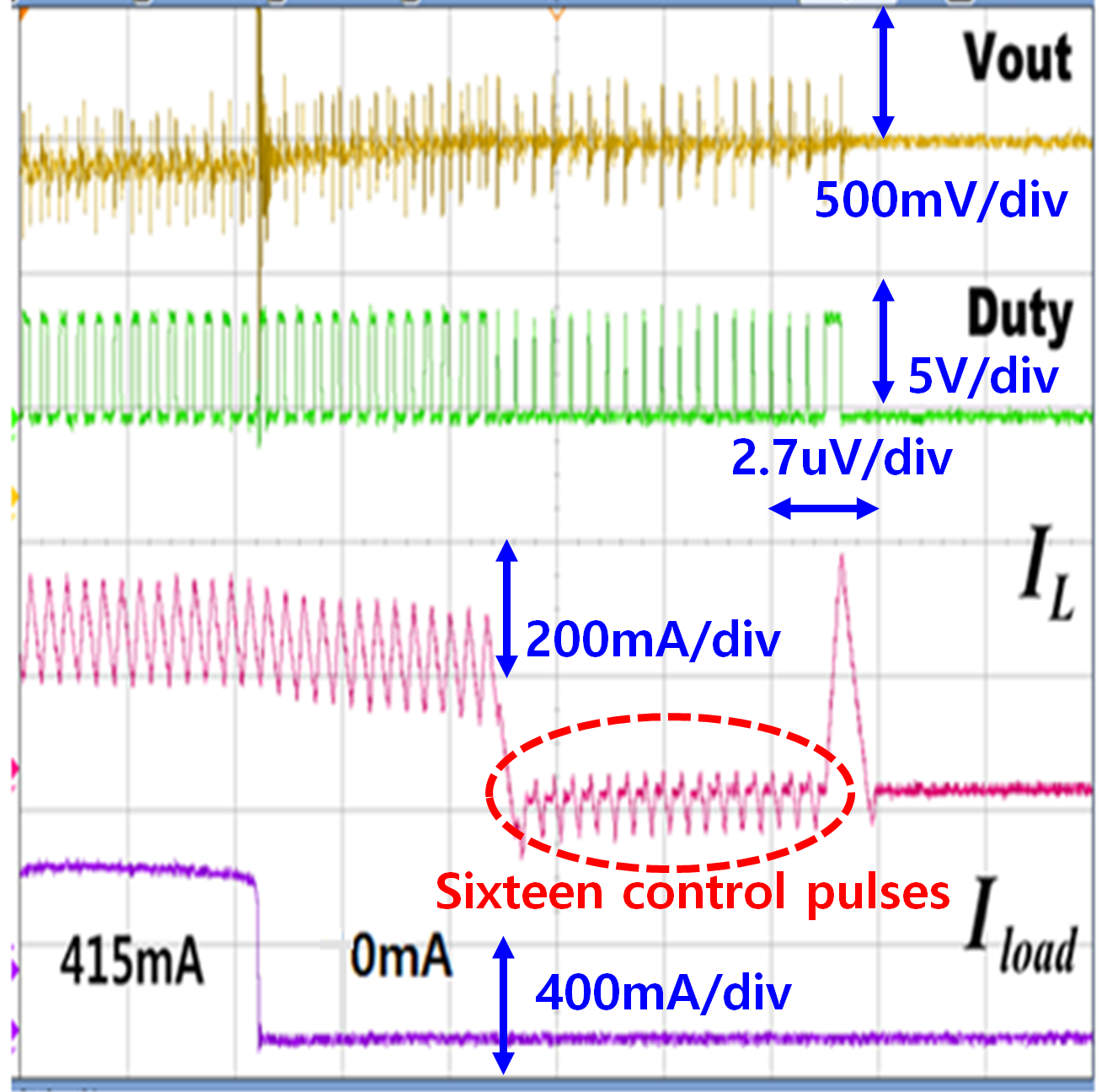##### Fig. 18. Measured power efficiency of the proposed dual- mode DC-DC converter as a function of the load current.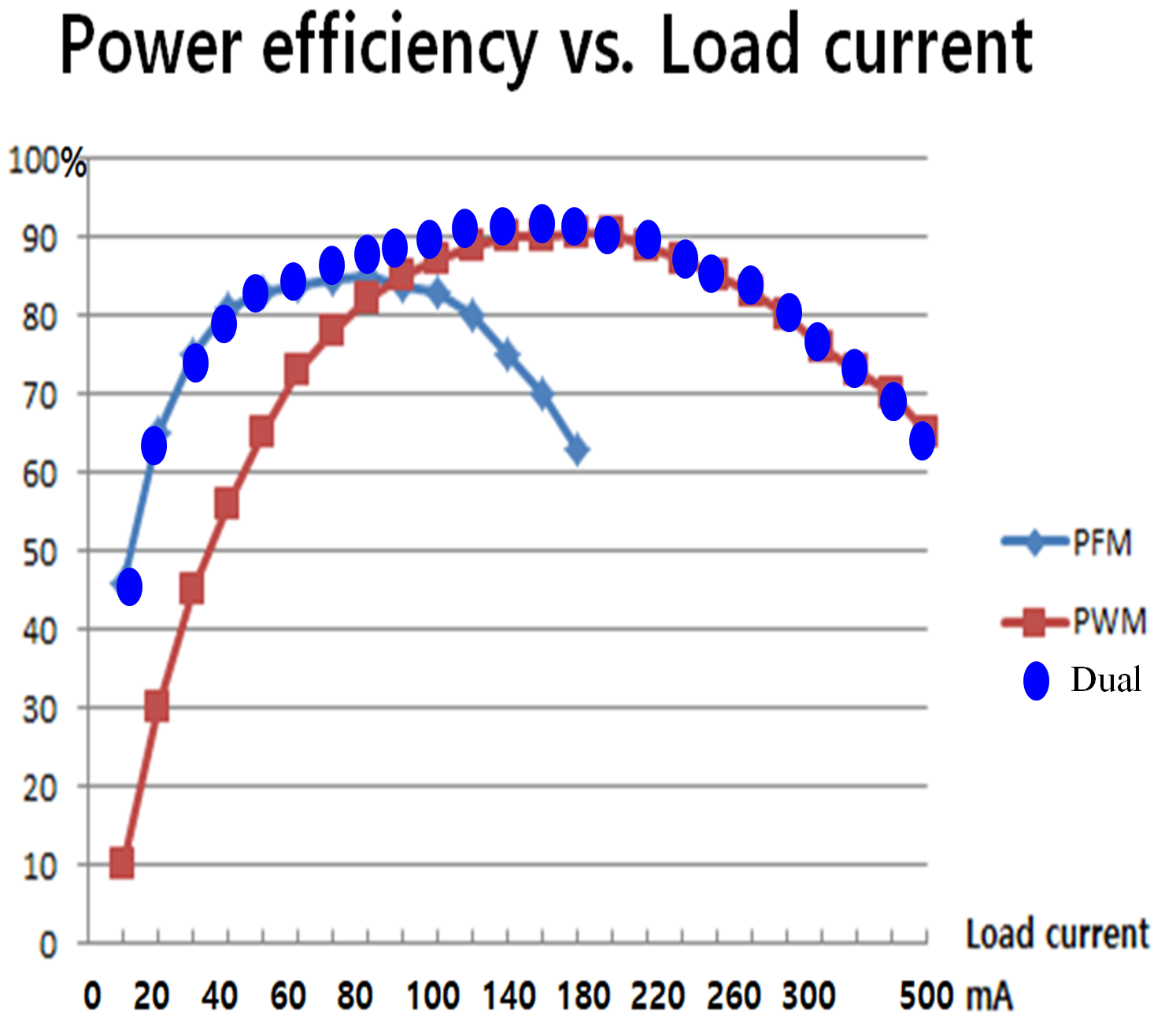## IV. CONCLUSIONS

This paper proposes a CMOS dual-mode DC-DC converter with the 3-bit digital mode controller. The proposed converter includes a PFM controller, a PWM controller, a PFM/PWM mode 3-bit controller, a dead time controller, gate drivers, a soft start circuit, and a bandgap reference within the on-chip. The dual-mode controller employed the 3-bit control circuit to select a number of counts from a 5-bit digital counter, so that the 3-bit control circuit was able to control the transient boundary load current between PFM and PWM modes and made a smooth transition between two modes. The proposed dual-mode DC-DC converter with a digital dual-mode controller was implemented through a 180 nm 1P/6M BCDMOS process. The active area of the DC-DC converter occupied 1760 ${\mu}$m x 1530 ${\mu}$m. The measurement results demonstrated the wide range of the input voltage from 2.2 V to 5.0 V with a fixed output voltage of 1.2 V. The proposed DC-DC converter achieved the peak PFM and PWM power efficiencies of 85% and 91 %, respectively, with an output ripple voltage of 12 - 32 mV at a switching frequency range of 60 kHz to 2.3 MHz and a wide load current range of 10~mA to 500 mA. If the analog compensation circuit is employed in the PWM controller, it may reduce the output ripple voltage with a heavy load current larger than 500 mA. The measurement results of the proposed dual-mode converter are comparable with those of the conventional ones. Especially the 3-bit digital control circuit allows the proposed circuit to change the boundary load current with bit control technique. Therefore, the proposed DC-DC converter can be employed by APs and MPUs that drive a wide range of output voltage and load current, and require a high power efficiency. If a number of digital-bit is extended beyond 3-bits, it will offer more degree of freedom to expand various boundary load current transient between PFM mode and PWM mode than that of the present proposed circuit.

### ACKNOWLEDGMENTS

This research was supported by Inha University Research Grant. The chip design was performed by Cadence Spectra design tool and the proposed chip was fabricated under sponsor of the IDEC MPW program. The authors thank to anonymous reviewers.

### References

1
Lee K., Kim H., Yoon J., Oh H., Park J. B., Park H., Lee Y., Dec. 2020, An Asynchronous Boost Converter With Time-Based Dual-Mode Control for Wide Load Range and High Efficiency in SSD Applications, IEEE Trans. Industrial Electronics, Vol. 67, No. 12, pp. 10520-105302
Sahu B., Rincon-Mora G., Feb. 2007, An Accurate, Low-Voltage, CMOS Switching Power Supply With Adaptive On-Time Pulse-Frequency Modulation (PFM) Control, IEEE Trans. Circuits and Systems I, Vol. 54, No. 2, pp. 312-3213
Chong J., So J., Yoon K., Feb. 2019, A CMOS PFM DC-DC converter employing a digitally programmable voltage level-shifting technique, Analog Integrated Circuits and Signal Processing, Vol. 98, No. 2, pp. 321-3294
Hwang Y., Chen J., Hou W., Liao P., Ku Y., Mar. 2016, A 10- μs Transient Recovery Time Low-EMI DC-DC DC-DC Converter With Δ–Σ Modulator, IEEE Trans. Very Large Scale Integration (VLSI) Systems, Vol. 24, No. 9, pp. 2983-29925
So J., Yang H., Park S., Lee J., Bae B., Yoon K., Nov. 2020, Three-level DC-DC converter utilizing a DAC-based flying capacitor voltage control technique, Analog Integrated Circuits and Signal Processing, Vol. 105, No. 2, pp. 321-3296
Erfani R., Marefat F., Mohseni P., Dec. 2020, A Dual-Output Single-Stage Regulating Rectifier With PWM and Dual-Mode PFM Control for Wireless Powering of Biomedical Implants, IEEE Trans. Biomed. Circuit and System, Vol. 14, No. 6, pp. 1195-12067
Noh Y., Seo J., Kim H., Lee S., 2022, A Reconfigurable DC-DC Converter for Maximum Thermoelectric Energy Harvesting in a Battery-Powered Duty-Cycling Wireless Sensor Node, IEEE J. Solid-State Circuits, pp. 1-128
Lee C. F., Mok P. K. T., Jan. 2004, A monolithic current-mode CMOS DC-DC converter with on-chip current sensing techniques, IEEE J. Solid-State Circuits, Vol. 39, No. 1, pp. 3-149
Ma F., Chen W., Wu J., Sep. 2007, A monolithic current-mode DC-DC converter with advanced control and protection circuits, IEEE Trans. Power Electron., Vol. 22, No. 5, pp. 1836-184610
Liou J., Yeh M., Kuo Y., 2008, A High Efficiency Dual-Mode DC-DC Converter IC For Portal Applications, IEEE Trans. Power Electron., Vol. 23, No. 2, pp. 667-67711
Xu W., Li Y., Gong X., Hong Z., Killat D., Mar. 2010, A Dual-Mode Single-Inductor Dual-Output Switching Converter With Small Ripple, IEEE Trans. Power Electron., Vol. 2512
Hong W., Lee M., Dec. 2020, A 7.4-MHz Tri-Mode DC-DC Buck Converter With Load Current Prediction Scheme and Seamless Mode Transition for IoT Applications, IEEE Trans. Circuits and Systems I, Vol. 67, No. 12, pp. 4544-455513
Kim S., Choi W., Podgurski R., Hanumolu P., Mar. 2018, A 10-MHz 2-800-mA 0.5-1.5-V 90% Peak Efficiency Time-Based Buck Converter With Seamless Transition Between PWM/PFM Modes, IEEE J. Solid-State Circuits, Vol. 53, No. 3, pp. 814-82414
Chen P., Wu C., Feb. 2016, A 50 nW-to-10mW Output Power Tri-Mode Digital Buck Converter With Self-Tracking Zero Current Detection for Photovoltaic Energy Harvesting, IEEE J. Solid-State Circuits, Vol. 51, No. 2, pp. 523-53215
Park Y., Park J., Kim H., Ryu H., Kim S., Pu Y., Hwang K., Yang Y., Lee M., Lee K., Sep. 2017, A Design of a 92.4% Efficiency Triple Mode Control DC-DC Buck Converter With Low Power Retention Mode and Adaptive Zero Current Detector for IoT/Wearable Application, IEEE Trans. Power Electron., Vol. 32, No. 9, pp. 6946-696016
Zhao M., Li M., Song S., Hu Y., Yao Y., bai X., Hu R., Wu X., Tan Z., Jan. 2022, An Ultra-Low Quiescent Current Tri-Mode DC-DC Buck Converter With 92.1% Peak Efficiency for IoT Applications, IEEE Trans. Circuits and Systems I, Vol. 69, No. 1, pp. 428-439## 저자소개

1991：BS degree in Electronic Engineering, Inha University, Incheon, Korea

1993：MS degree in Electronic Engineering, Inha University, Incheon, Korea

2003：PhD degree in University of Florida, Gainesville, USA

From 2003 to 2016, he was with Samsung Display Inc., Korea, as a Principal Research Engineer, where he worked on semiconductor and display devices design and development. In 2017, he joined the Department of System Semiconductor Engineering, Sangmyung University, Korea, where he is currently a Professor. His current research interests include a mixed-signal circuit and device modeling and simulation, physics-based artificial neural network, noise modeling in nanoscale MOSFETs, display device design, and thermoelectric transport modeling.

Kwang Sub Yoon received BS degree in EE from Inha University in 1981, MS, and Ph.D. degrees in EE from Georgia Institute of Technology in 1983 and 1990, respectively. He worked at Silicon Systems Inc. (Tustin, Calif.) as a senior design engineer for 1988-1992. Since 1992, he joined the department of Electronic Engineering at Inha University, Korea. He established IEEE ED/SSC joint chapter in 1998 and served as chairman until 2016. He served as TPC chairman and general chairman of ISOCC (International SoC Conference) for 2012 and 2013, respectively. He also serves as associate editor of JSTS (Journal of Semiconductor Technology and Science) and associate editor of JICAS, IDEC. His research interests include mixed-signal CMOS circuit design such as high performance data converters (high speed(flash-pipeline, time-interleaving), high resolutions(sigma-delta), low power(SAR) ADCs, hybrid ADCs, and DACs), PLLs, PMICs (Buck(PFM-PWM)/Boost) for IoTs, Smart sensor systems, and Bio-signal processing.